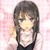## FANDOM

25,790 Pages# Arrogant Schmuck

0 Discussion posts
My favorite wikis
• I live in the basement where I keep all my [DATA EXPUNGED]
• I was born on August 25
• My occupation is Clout Hunter
• I am Despacito
•## VeggieTales: LarryBoy Calcs

September 19, 2019 by Arrogant Schmuck

Here are some calcs for the Cucumber of Steel.

I'm going to be treating Larry as the size of an average human. In the Larry Boy segments, the veggies are the same size compared to real life animals like whales and penguins that humans are.

Feat here

(928/642)*1.77 = 2.5585046729 meters

(136/298)*2.5585046729 = 1.16763971649 meters

(1059/230)*1.16763971649 = 5.37621939027 meters

(130/177)*5.37621939027 = 3.94863571037 meters

(692/177)*5.37621939027 = 21.0188916275 meters

There's no angle which shows the width; for right now I'll say it's half the length. No particular reason, in all honesty.

21.0188916275*10.5094458137*3.94863571037 = 872.241398008 m^3

It takes 32 of these plungers to lift this while Larry has two in his suit

872.241398008/16 = 54.515…

•## Shrek: Dragon Destroys Bridge

September 9, 2019 by Arrogant Schmuck

The dragon destroys part of a bridge with just its tail.

Calc time!

Shrek is 7 feet tall (2.1336 meters)

(451/410)*2.1336 = 2.34696 meters

Angular size gives me 6.0484 meters for the length of the part of the bridge that was destroyed.

(119/133)*2.34696 = 2.09991157895 meters for the width of the part of the bridge that was destroyed

(26/271)*6.0484 = 0.58028929889 meters for the thickness

((19/271)*6.0484)/2 = 0.21202878228 meters for radius of the column

(110/271)*6.0484 = 2.4550701107 meters for the height of the column

(37/271)*6.0484 = 0.82579630996 meters for this part of the bridge Idk what to call

(91/271)*6.0484 = 2.03101254613 meters for this part of the bridge Idk what to call

6.0484*2.09991157895*0.58028929889 = 7.37031542822 m^3

0.825796309…

•## COOLverse: Singularity and Interplanetary Travel

September 1, 2019 by Arrogant Schmuck

Matt told me about this series, Dioti Manual ~Kamisamatachi no Ren'ai Daikou~, a while back and I've been meaning to get these two feats out there.

According to this calc the average human head is 23.9 cm or 0.239 meters

(57/207)*0.239 = 0.0658115942 meters

Using the method used in this calc

Black Hole Radius = 0.0329057971 meters

Black Hole Mass = (c² * radius) / (2 * G)

Black Hole Mass = ((299792458^2) * 0.0329057971) / (2 * (6.67408e-11)) = 2.2156054e+25 Kg

Black Hole Solar Mass = (2.2156054e+25 / 1.98855e+30) = 0.00001114181

Energy to create that Black Hole = 0.00001114181 * 5.693e+41 Joules = 6.3430324e+36 joules or 1.516 ninatons of TNT or Large Planet level

These things travel from the Laniakea Supercluster to Earth

That supercluster is 251.1 m…

•## Jimmy and Timmy Power Hour 3 Calcs

August 14, 2019 by Arrogant Schmuck

Some of y'all may remember there was a series of three specials where FOP and Jimmy Neutron crossed over. Well I'm going to be calcing feats from the third just cuz I need to in order to make a profile.

Jimmy and Timmy dodge these lasers

Timmy is 4'2 or 1.27 meters tall

(294/449)*1.27 = 0.83158129175 meters

(1456/449)*1.27 = 4.11830734967 meters

(0.83158129175*299792458) / (4.11830734967) = 60535015.5569 m/s or 0.2c or Relativistic

They smack this guy all the way from Dimmsdale to London

Dimmsdale is in California

California and London are 8558 km apart

He flies there in about 6 seconds

8558 km/6 = 1426333.3333300001 m/s

And let's assume this guy is on the heavier side since he's part metal and taller than average.

I'll go with 154 kg

(154*0.5)*(1426333…

•## ChalkZone: Rudy's Got the Feats!

August 10, 2019 by Arrogant Schmuck

After watching through all of ChalkZone, here are some calcs for your reading pleasure.

The Beanie Boys get hit by this large, metal ball.

Now Snap has no official height, but if you watch the series, he is definitely on the short side. So I'll say he's the height of the average 10 year-old boy.

Average 10 year-old boy is 138.4 cm tall

(792/431)*1.384 = 2.54322041763

(464/84)*2.54322041763 = 14.0482651641

Volume of 1451.67

Steel has density of 8050

1451.67*8050 = 11685943.5 kg

Angsizing gives me a distance of 68.57 meters

It takes about a second for the sphere to get from that point to where it hits the Beanie Boys.

(11685943.5*0.5)*(68.57^2) = 27472746923.6 joules or 6.5661441021988525 tons of TNT or Large Building level+

Rudy gets launched right smac…